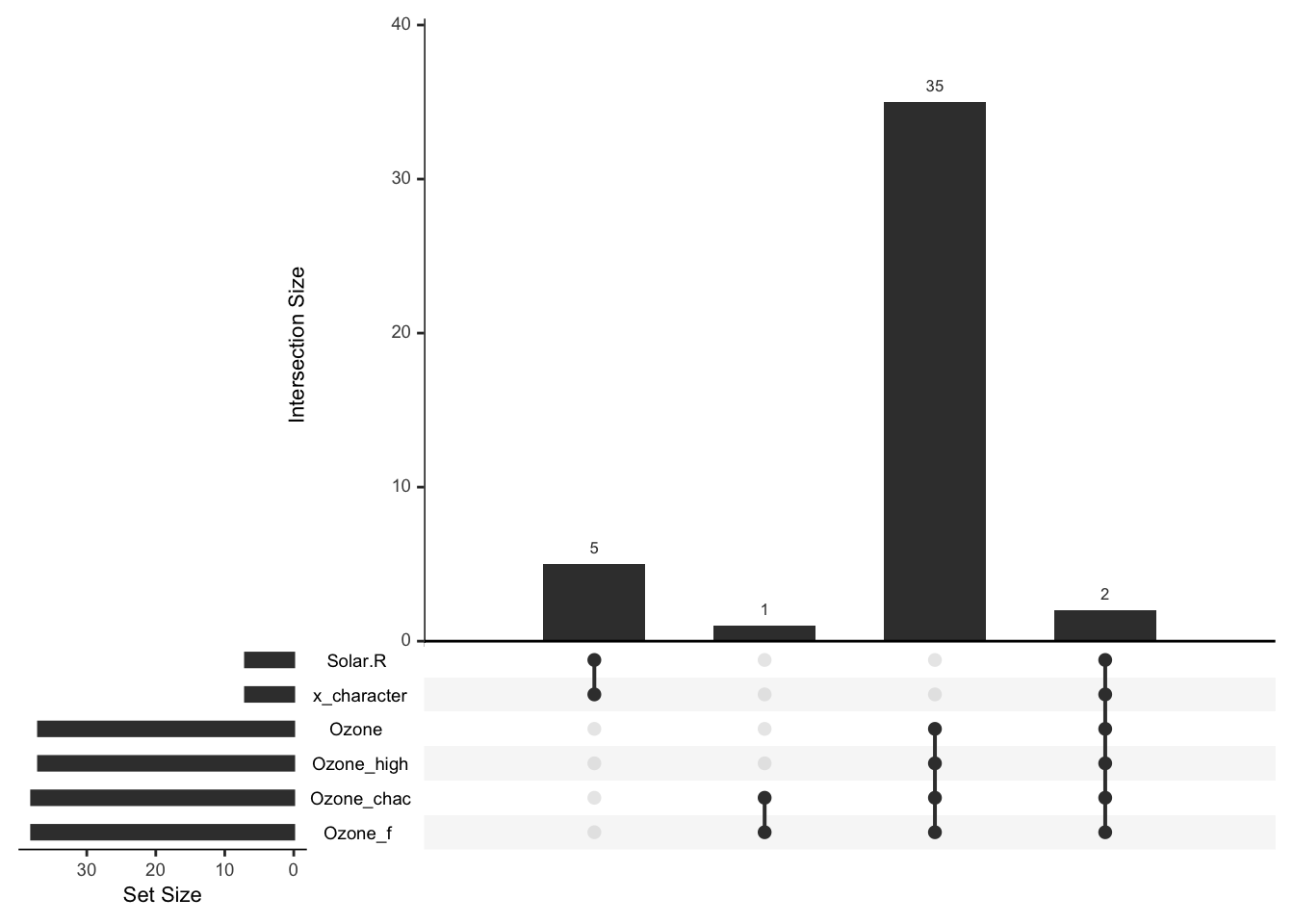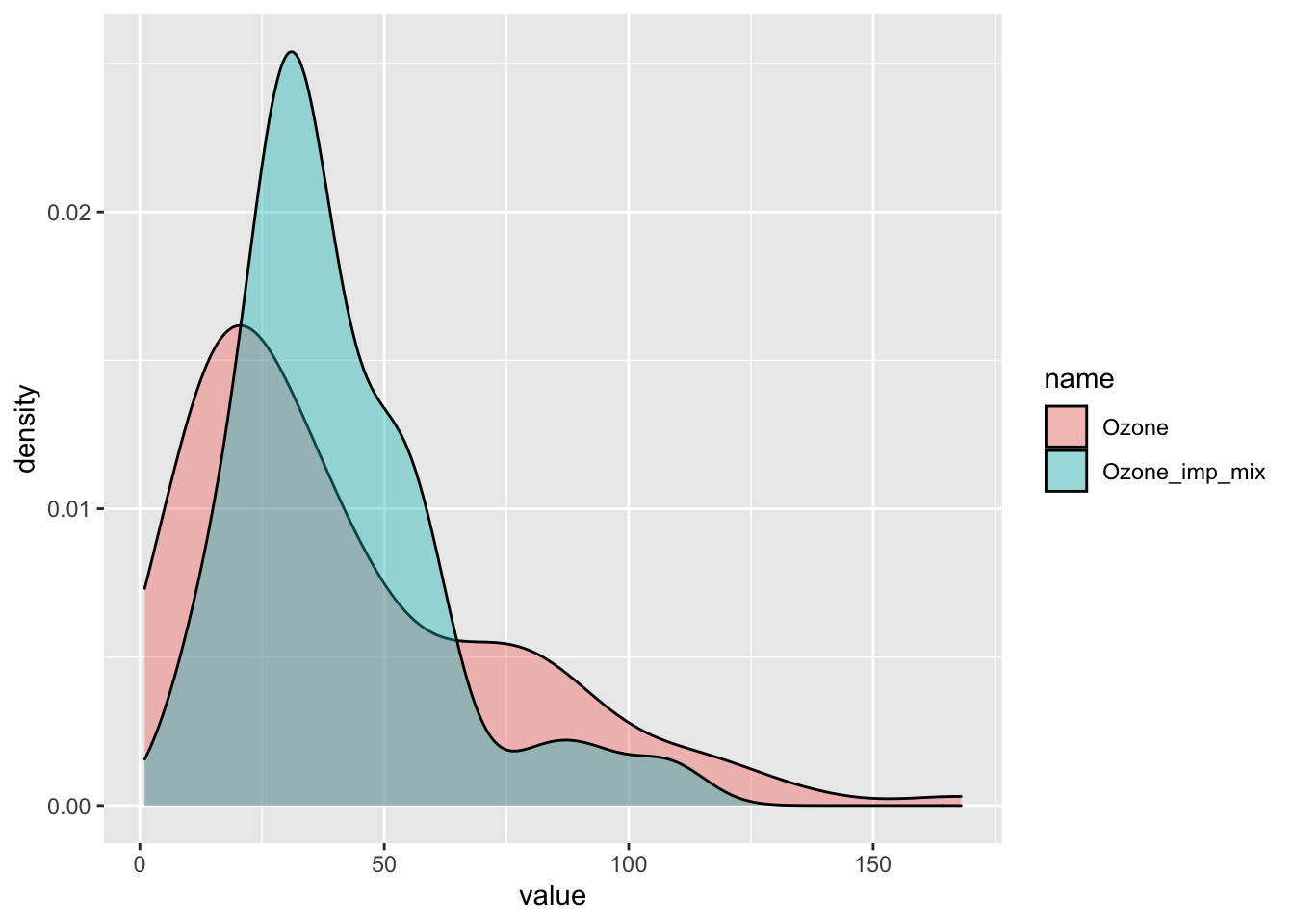``````pkgs <- c("miceFast", "mice", "car", "ggplot2", "dplyr", "data.table")
inst <- lapply(pkgs, library, character.only = TRUE)``````
``set.seed(123456)``

## Description

Fast imputations under the object-oriented programming paradigm. There was used quantitative models with a closed-form solution. Thus package is based on linear algebra operations. The biggest improvement in time performance could be achieve for a calculation where a grouping variable have to be used. A single evaluation of a quantitative model for the multiple imputations is another major enhancement. Moreover there are offered a few functions built to work with popular R packages.

## Performance

miceFast was compared1 with the mice package. For grouping option there was used a basic R looping and popular dplyr/data.table packages. Summing up, miceFast offer a relevant reduction of a calculations time for:

• Linear Discriminant Analysis around (x5)
• where a grouping variable have to be used (around x10 depending on data dimensions and number of groups and even more than x100 although compared to data.table only a few k faster or even the same) because of pre-sorting by grouping variable
• multiple imputations is faster around x(a number of multiple imputations) because the core of a model is evaluated only ones.
• Variance inflation factors (VIF) (x5) because the unnecessary linear regression is not evaluated - we need only inverse of X’X
• Predictive mean matching (PMM) (x100) because of pre-sorting and binary search If you are interested about the procedure of testing performance check performance_validity.R file at the extdata folder.
``system.file("extdata", "performance_validity.R", package = "miceFast")``

Additional plots for simulations with certain parameters (but feel free to change them) are located:

``system.file("extdata", "images", package = "miceFast")``

Moreover there are offered a few functions built to work with the popular R packages such as ‘data.table’.

## Motivations

Missing data is a common problem. The easiest solution is to delete observations for which a certain variable is missing. However this will somek deteriorate quality of a project. Another solution will be to use methods such as multiple/regular imputations to fill the missing data. Non missing independent variables could be used to approximate a missing observations for a dependent variable. R or Python language are user-friendly for data manipulation but parallely brings slower computations. Languages such as C++ gives an opportunity to boost our applications or projects.

## Introduction for data.table/dplyr users - using additional functions from miceFast:

Usage of `fill_NA` and `fill_NA_N` functions from miceFast - this functions should be resistant to glitches from an user activity perspective and a data structure.

### Data

``````# airquality dataset with additional variables
data(air_miss)``````

NA structure

``upset_NA(air_miss, 6)``### Intro: data.table

``````# VIF - values bigger than 10 (around) suggest that there might be a collinearity problem.
# VIF is high for Solar.R and x_character which is obvious - x_character is a factor version of numeric Solar.R
air_miss[, .(VIF(.SD,
posit_y = "Ozone",
posit_x = c(
"Solar.R",
"Wind",
"Temp",
"x_character",
"Day",
"weights",
"groups"
)
))]``````
``````##           V1
## 1: 24.978996
## 2:  1.445308
## 3:  3.077776
## 4: 42.248792
## 5:  1.083795
## 6:  1.100853
## 7:  2.954588``````
``````# IMPUTATIONS
# Imputations with a grouping option (models are separately assessed for each group)
# taking into account provided weights
air_miss[, Solar_R_imp := fill_NA_N(
x = .SD,
model = "lm_bayes",
posit_y = "Solar.R",
posit_x = c("Wind", "Temp", "Intercept"),
w = .SD[["weights"]],
k = 100
), by = .(groups)] %>%
# Imputations - discrete variable
.[, x_character_imp := fill_NA(
x = .SD,
model = "lda",
posit_y = "x_character",
posit_x = c("Wind", "Temp", "groups")
)] %>%
# logreg was used because almost log-normal distribution of Ozone
# imputations around mean
.[, Ozone_imp1 := fill_NA(
x = .SD,
model = "lm_bayes",
posit_y = "Ozone",
posit_x = c("Intercept"),
logreg = TRUE
)] %>%
# imputations using positions - Intercept, Temp
.[, Ozone_imp2 := fill_NA(
x = .SD,
model = "lm_bayes",
posit_y = 1,
posit_x = c(4, 6),
logreg = TRUE
)] %>%
# model with a factor independent variable
# multiple imputations (average of x30 imputations)
# with a factor independent variable, weights and logreg options
.[, Ozone_imp3 := fill_NA_N(
x = .SD,
model = "lm_noise",
posit_y = "Ozone",
posit_x = c("Intercept", "x_character_imp", "Wind", "Temp"),
w = .SD[["weights"]],
logreg = TRUE,
k = 30
)] %>%
.[, Ozone_imp4 := fill_NA_N(
x = .SD,
model = "lm_bayes",
posit_y = "Ozone",
posit_x = c("Intercept", "x_character_imp", "Wind", "Temp"),
w = .SD[["weights"]],
logreg = TRUE,
k = 30
)] %>%
.[, Ozone_imp5 := fill_NA(
x = .SD,
model = "lm_pred",
posit_y = "Ozone",
posit_x = c("Intercept", "x_character_imp", "Wind", "Temp"),
w = .SD[["weights"]],
logreg = TRUE
), .(groups)] %>%
.[, Ozone_imp6 := fill_NA_N(
x = .SD,
model = "pmm",
posit_y = "Ozone",
posit_x = c("Intercept", "x_character_imp", "Wind", "Temp"),
w = .SD[["weights"]],
logreg = TRUE,
k = 10
), .(groups)] %>%

# Average of a few methods
.[, Ozone_imp_mix := apply(.SD, 1, mean), .SDcols = Ozone_imp1:Ozone_imp6] %>%

# Protecting against collinearity or low number of observations - across small groups
# Be carful when using a data.table grouping option
# because of lack of protection against collinearity or low number of observations.
# There could be used a tryCatch(fill_NA(...),error=function(e) return(...))

.[, Ozone_chac_imp := tryCatch(fill_NA(
x = .SD,
model = "lda",
posit_y = "Ozone_chac",
posit_x = c("Intercept", "Month", "Day", "Temp", "x_character_imp"),
w = .SD[["weights"]]
),
error = function(e) .SD[["Ozone_chac"]]
), .(groups)]``````

### Intro: dplyr

``````# VIF - values bigger than 10 (around) suggest that there might be a collinearity problem.
# VIF is high for Solar.R and x_character which is obvious - x_character is a factor version of numeric Solar.R
air_miss %>%
do(vifs = VIF(.,
posit_y = "Ozone",
posit_x = c(
"Solar.R",
"Wind",
"Temp",
"x_character",
"Day",
"weights",
"groups"
)
)) %>%
unlist()``````
``````##     vifs1     vifs2     vifs3     vifs4     vifs5     vifs6     vifs7
## 24.978996  1.445308  3.077776 42.248792  1.083795  1.100853  2.954588``````
``````# IMPUTATIONS
air_miss <- air_miss %>%
# Imputations with a grouping option (models are separately assessed for each group)
# taking into account provided weights
group_by(groups) %>%
do(mutate(., Solar_R_imp = fill_NA(
x = .,
model = "lm_pred",
posit_y = "Solar.R",
posit_x = c("Wind", "Temp", "Intercept"),
w = .[["weights"]]
))) %>%
ungroup() %>%
# Imputations - discrete variable
mutate(x_character_imp = fill_NA(
x = .,
model = "lda",
posit_y = "x_character",
posit_x = c("Wind", "Temp")
)) %>%
# logreg was used because almost log-normal distribution of Ozone
# imputations around mean
mutate(Ozone_imp1 = fill_NA(
x = .,
model = "lm_bayes",
posit_y = "Ozone",
posit_x = c("Intercept"),
logreg = TRUE
)) %>%
# imputations using positions - Intercept, Temp
mutate(Ozone_imp2 = fill_NA(
x = .,
model = "lm_bayes",
posit_y = 1,
posit_x = c(4, 6),
logreg = TRUE
)) %>%
# multiple imputations (average of x30 imputations)
# with a factor independent variable, weights and logreg options
mutate(Ozone_imp3 = fill_NA_N(
x = .,
model = "lm_noise",
posit_y = "Ozone",
posit_x = c("Intercept", "x_character_imp", "Wind", "Temp"),
w = .[["weights"]],
logreg = TRUE,
k = 30
)) %>%
mutate(Ozone_imp4 = fill_NA_N(
x = .,
model = "lm_bayes",
posit_y = "Ozone",
posit_x = c("Intercept", "x_character_imp", "Wind", "Temp"),
w = .[["weights"]],
logreg = TRUE,
k = 30
)) %>%
group_by(groups) %>%
do(mutate(., Ozone_imp5 = fill_NA(
x = .,
model = "lm_pred",
posit_y = "Ozone",
posit_x = c("Intercept", "x_character_imp", "Wind", "Temp"),
w = .[["weights"]],
logreg = TRUE
))) %>%
do(mutate(., Ozone_imp6 = fill_NA_N(
x = .,
model = "pmm",
posit_y = "Ozone",
posit_x = c("Intercept", "x_character_imp", "Wind", "Temp"),
w = .[["weights"]],
logreg = TRUE,
k = 20
))) %>%
ungroup() %>%
# Average of a few methods
mutate(Ozone_imp_mix = rowMeans(select(., starts_with("Ozone_imp")))) %>%

# Protecting against collinearity or low number of observations - across small groups
# Be carful when using a data.table grouping option
# because of lack of protection against collinearity or low number of observations.
# There could be used a tryCatch(fill_NA(...),error=function(e) return(...))
group_by(groups) %>%
do(mutate(., Ozone_chac_imp = tryCatch(fill_NA(
x = .,
model = "lda",
posit_y = "Ozone_chac",
posit_x = c("Intercept", "Month", "Day", "Temp", "x_character_imp"),
w = .[["weights"]]
),
error = function(e) .[["Ozone_chac"]]
))) %>%
ungroup()``````

### results - visualization

``````# Distribution of imputations vs Distribution of initial data
compare_imp(air_miss, origin = "Ozone", target = "Ozone_imp_mix")````````````# or
compare_imp(air_miss, origin = "Ozone", target = c("Ozone_imp2", "Ozone_imp_mix"))``````